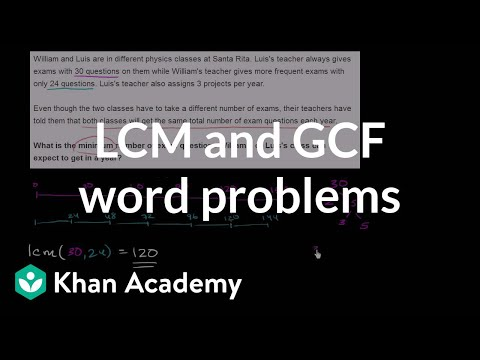# Least common multiple: repeating factors (Full video)

Description: Sal finds the LCM (least common multiple) of 25 and 30. Created by Sal Khan. We need to figure out the least common multiple of 30 and 25. And I'm going to do this using the prime factorization method which I just like more. Let's find the prime factorization of both of these numbers.

### Other videos you might be interested in### Developing strategies for multiplying decimals (Full video)